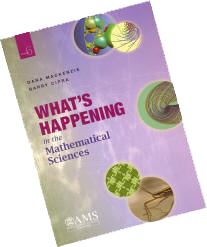Select Page

# WHIMS Volume 6What’s Happening in the Mathematical Sciences, Vol. 6 covers roughly the period from 2000 to 2006, and it was co-written by Barry Cipra and me. Here is an annotated version of the table of contents.

1. First of Seven Millennium Problems Nears Completion (Cipra).

An update on Grigory Perelman’s proof of the Poincaré Conjecture, one of seven problems that had been given \$1 million bounties by the Clay Mathematics Foundation in 2000.

Branch of mathematics: Geometry, topology.

2. Classifying Hyperbolic Manifolds — All’s Well That Ends Well.

What were topologists doing while they awaited definitive word on the Poincaré Conjecture? Well, some of them were solving a series of long-standing conjectures about the ends of hyperbolic (negatively-curved) spaces.

Branch of mathematics: Topology.

3. Digits of Pi (Cipra).

Pi lovers rejoice! You can now compute the trillionth digit of pi without having to compute all the preceding ones. The only catch is that you have to use hexadecimal notation (base-16, the same as computers).

Branch of mathematics: Analysis (a.k.a. calculus), computer science.

4. Combinatoricists Solve a Venn-erable Problem (Cipra).

A question posed by an undergraduate student — can a Venn diagram be rotationally symmetric? — leads to some beautiful “doilies,” intricate mathematics, and (after forty years) a solution found by another student.

Branch of mathematics: Combinatorics.

5. New Insights Into Prime Numbers.

This chapter covers three remarkable developments in number theory, including a super-efficient way to test large numbers for primality and two new insights into the large-scale distribution of primes.

Branch of mathematics: Number theory.

6. From Rubik’s Cube to Quadratic Number Fields … and Beyond.

Inspired by a famous toy, Manjul Bhargava re-interprets a mysterious operation called “Gauss composition” in terms of operations on a box. This results in a whole series of results on previously unassailable problems in number theory, such as the number of cubic, quartic, or quintic number fields of a given discriminant.

Branch of mathematics: Number theory.

7. Vortices in the Navier-Stokes Equations (Cipra).

What keeps Jupiter’s Giant Red Spot going? At least for an idealized model of fluid flow, mathematicians have managed to prove the existence of vortices that last forever.

Branch of mathematics: Differential equations.

8. Fluid Dynamics Explains Mysteries of Insect Motion.

For insects, the hard part of walking on water isn’t staying afloat — it’s moving forward when they have nothing to push against. How do they do it? Water striders, as well as several other insects (such as dragonflies), use vortices of water or air to accomplish seemingly impossible feats.

Branch of mathematics: Mathematical biology, applied mathematics, differential equations.

9. Brownian Motion, Phase Transitions, and Conformal Maps.

In the early 2000s a huge leap forward took place in the understanding of random processes, like the jitters of air molecules or the percolation of water through soil. The late Oded Schramm discovered a sort of universal template that explains which processes are “jitterier” than others and provides a methodology for making predictions.

Branch of mathematics: Probability and statistics, mathematical physics.

10. Smooth(ed) Moves(Cipra).

Using a new measure of complexity, Dan Spielman and Shang-Hua Teng explain why certain algorithms of computer science (such as the simplex method for linear programming problems) almost always work better than they are supposed to.

Branch of mathematics: Computer science.

Cover images (from top): Fundamental domain of a Kleinian group (see Chapter 2); the Burgers vortex (see Chapter 7); lamination of a torus (see Chapter 2); tessellation of the hyperbolic plane (see Chapter 2); a water strider uses surface tension to stay afloat, but how does he move forward? (See Chapter 8.)